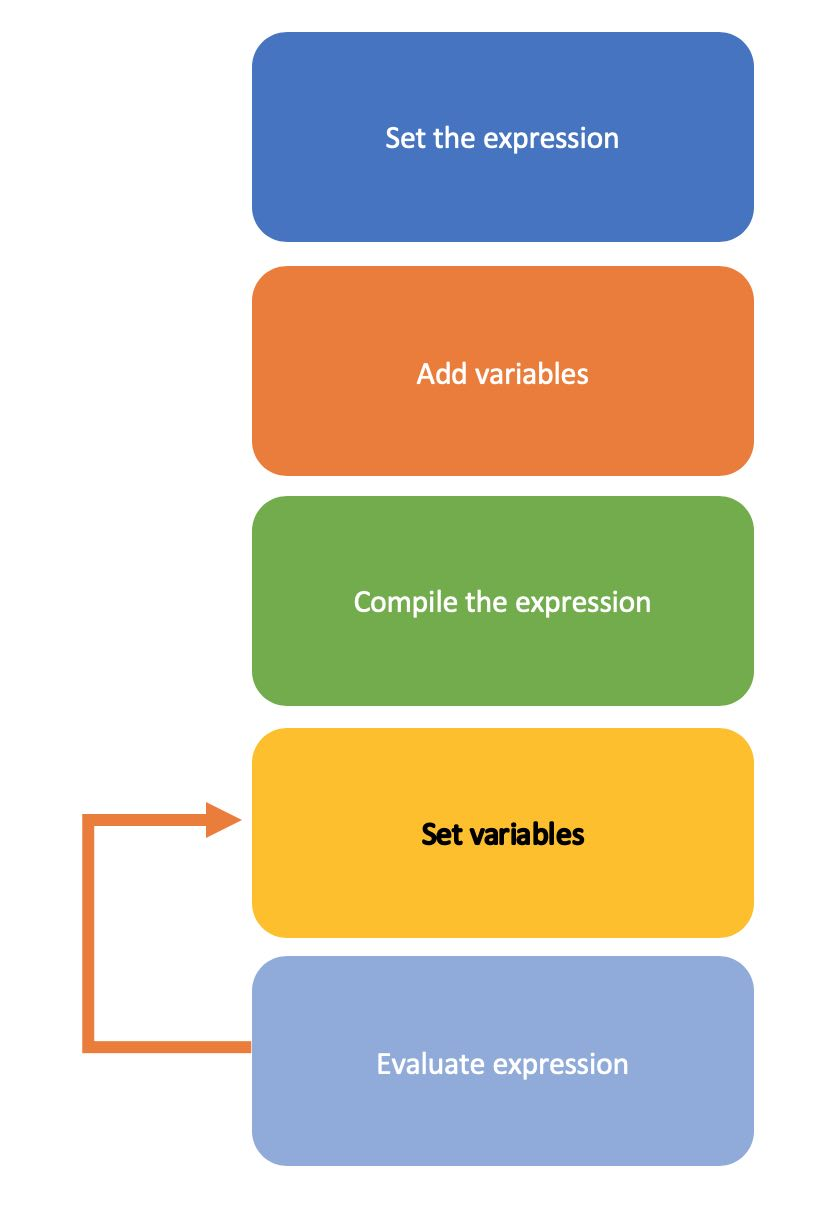Jamie Balfour

Welcome to my personal website.

Find out more about me, my personal projects, reviews, courses and much more here.

# Official ZPE/YASS documentationCalculator object

## Introduction

The Calculator object was added in version 1.8.7 (Portman).

The premise behind the Calculator object is to allow users to write a formula once (an expression) compile it, and then simply set variables on the calculator object and finally evaluate it, making it much quicker than using standard maths. This is demonstrated below:This diagram shows the processes involved in the use of the Calculator object.

The Calculator object is incredibly efficient because it takes advantage of the Zenith Parsing Engine to perform parsing and Z Compilation to compile the code into an abstract syntax tree. One should only need to compile the expression once the expression is changed.

## Calculator object functions

The following is a list of internal functions the Calculator object exposes. All functions are ZPEObjectNativeFunctions unless specified therefore run in native code.

add_variable(string id) ⇒ boolean
Adds a variable to the list of variables.
set_*() ⇒ boolean
Sets a variable that has already been declared. The * in the name is the name of the variable, for example if a variable p has been declared the name of this function is set_p.
set_expression(string expr) ⇒ boolean
Sets the internal expression.
compile() ⇒ boolean
Attempts to compile the expression.
evaluate() ⇒ mixed
Evaluates the compiled expression to a real. If the expression has not been compiled it will return false.

## Examples

The first example is my favourite formula of all, the power rating of a CMOS transistor at some frequency:

$$P = CV^2af$$

This can be written within our program and compiled using the Calculator:

YASS
$calc = new Calculator()$calc->set_expression("C * V^2 * a * f")

$calc->add_variable("C")$calc->add_variable("V")
$calc->add_variable("a")$calc->add_variable("f")

$calc->compile()$calc->set_C(0.000006)
$calc->set_V(1.25)$calc->set_a(0.91)
$calc->set_f(1250E6) print($calc->evaluate())

$calc->set_f(2255E6) print($calc->evaluate())


This next example is Ohm's law:

$$V = IR$$

This can be written within our program and compiled using the Calculator:

YASS
$calc = new Calculator()$calc->set_expression("I * R")

$calc->add_variable("I")$calc->add_variable("R")

$calc->compile()$calc->set_I(12.0)
$calc->set_R(3.0) print($calc->evaluate())

$calc->set_I(2.3) print($calc->evaluate())


This last example is used to calculate the electrical power consumption of a device

$$P = IV$$

This can be written within our program and compiled using the Calculator:

YASS
$calc = new Calculator()$calc->set_expression("I * V")

$calc->add_variable("I")$calc->add_variable("V")

$calc->compile()$calc->set_I(2.5)
$calc->set_V(12.0) print($calc->evaluate())

$calc->set_I(3.0) print($calc->evaluate())


Other expressions worth testing include (most of these come from another pet love I have in Physics):

• $$Q = It$$
• $$E = \frac{1}{2}QV$$
• $$s = ut + \frac{1}{2}at^2$$
• $$E_k = \frac{1}{2}mv^2$$
• $$v = f\lambda$$